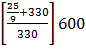# A musician suing an open flute of length 50 cm produces second harmonic sound waves.  A persons runs towards the musician from another end of a hall at a speed of 10km/h.  If the wave speed is 330 m/s, the frequency heard by the running person shall be close to a. 753 Hz b. 500 Hz   333 Hz d. 666 Hz

## Question ID - 50119 :- A musician suing an open flute of length 50 cm produces second harmonic sound waves.  A persons runs towards the musician from another end of a hall at a speed of 10km/h.  If the wave speed is 330 m/s, the frequency heard by the running person shall be close to a. 753 Hz b. 500 Hz   333 Hz d. 666 Hz

3537

Frequency of the sound produced by flute,

f = 2Hz

Velocity of observer, v0 = 10×∴ Frequency detected by observer, f =∴ f== 335.56×2 = 671.12

 An equilateral triangle is described on the diagonal of a square. What is the ratio of the area of the triangle to that of the square? (a) 2:(b) 4:(c):2 (d):4